### Open Methods: Secant Method

### The Method

The secant method is an alternative to the Newton-Raphson method by replacing the derivative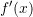with its finite-difference approximation. The secant method thus does not require the use of derivatives especially when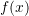is not explicitly defined. In certain situations, the secant method is preferable over the Newton-Raphson method even though its rate of convergence is slightly less than that of the Newton-Raphson method. Consider the problem of finding the root of the function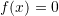. Starting with the Newton-Raphson equation and utilizing the following approximation for the derivative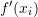: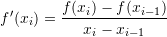the estimate for iteration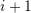can be computed as: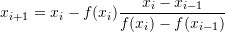Obviously, the secant method requires two initial guesses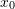and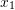.

#### Example

As an example, let’s consider the function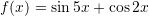. Setting the maximum number of iterations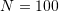,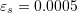,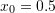, and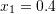, the following is the Microsoft Excel table produced: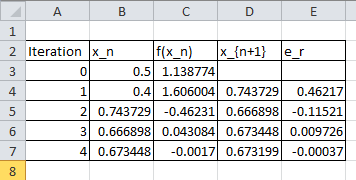The Mathematica code below can be used to program the secant method with the following output: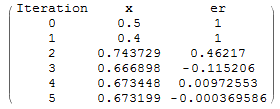View Mathematica Code
f[x_] := Sin[5 x] + Cos[2 x];
xtable = {0.5, 0.4};
er = {1,1};
es = 0.0005;
MaxIter = 100;
i = 2;
While[And[i <= MaxIter, Abs[er[[i]]] > es], xnew = xtable[[i]] - (f[xtable[[i]]]) (xtable[[i]] - xtable[[i - 1]])/(f[xtable[[i]]] -f[xtable[[i - 1]]]); xtable = Append[xtable, xnew];  ernew = (xnew - xtable[[i]])/xnew; er = Append[er, ernew]; i++];
T = Length[xtable];
SolutionTable = Table[{i - 1, xtable[[i]], er[[i]]}, {i, 1, T}];
SolutionTable1 = {"Iteration", "x", "er"};
T = Prepend[SolutionTable, SolutionTable1];
T // MatrixForm

View Python Code
import numpy as np
import pandas as pd

def f(x): return np.sin(5*x) + np.cos(2*x)
xtable = [0.5, 0.4]
er = [1,1]
es = 0.0005
MaxIter = 100
i = 1
while i <= MaxIter and abs(er[i]) > es:
xnew = xtable[i] - (f(xtable[i]))*(xtable[i] - xtable[i - 1])/(f(xtable[i]) -f(xtable[i - 1]))
xtable.append(xnew)
ernew = (xnew - xtable[i])/xnew
er.append(ernew)
i+=1
SolutionTable = [[i, xtable[i], er[i]] for i in range(len(xtable))]
pd.DataFrame(SolutionTable, columns=["Iteration", "x", "er"])


The following code runs the Secant method to find the root of a functionwith two initial guessesand. The value of the estimate and approximate relative error at each iteration is displayed in the command window. Additionally, two plots are produced to visualize how the iterations and the errors progress. The program waits for a keypress between each iteration to allow you to visualize the iterations in the figure. Call the function with secant(@(x) f(x), x0, x1). For example try secant(@(x) sin(5.*x)+cos(2.*x),0.5,0.4)

### Convergence Analysis of the Secant Method

Theestimate in the secant method is obtained as follows:Multiplying both sides by -1 and adding the true value of the root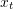where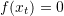for both sides yields: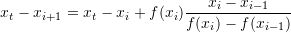Using algebraic manipulations: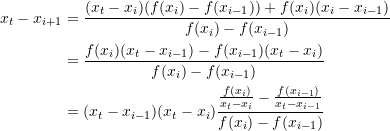Using the Mean Value Theorem, the denominator on the right-hand side can be replaced with: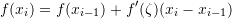for some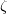between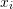and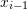. Therefore,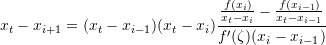Using Taylor’s theorem for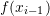and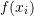aroundwe get: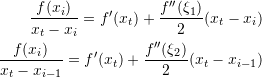for some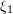betweenandand some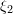betweenand. Using the above expressions we can reach the equation: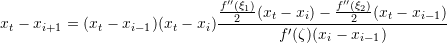andcan be assumed to be identical and equal to, therefore: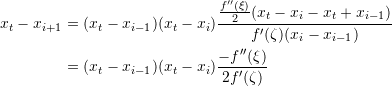Therefore:

(1)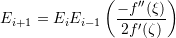Comparing the convergence equation of the Newton Raphson method with 1 shows that the convergence in the secant method is not quite quadratic. To find the order of convergence, we need to solve the following equation for a positive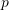and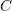: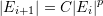Substituting into equation 1 yields: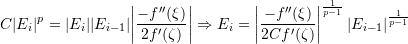But we also have: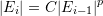Therefore: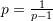. This equation is called the golden ratio and has the positive solution for: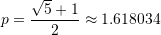while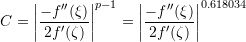implying that the error convergence is not quadratic but rather: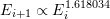The following tool visualizes how the secant method converges to the true solution using two initial guesses. Using,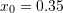,, and solving for the root of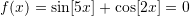yields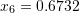.

The following Mathematica Code was utilized to produce the above tool:

View Mathematica Code
Manipulate[
f[x_] := x^3 - x + 3;
f[x_] := Sin[5 x] + Cos[2 x];
xtable = {-0.5, -0.6};
xtable = {0.35, 0.4};
er = {1, 1};
es = 0.0005;
MaxIter = 100;
i = 2;
While[And[i <= MaxIter, Abs[er[[i]]] > es], xnew = xtable[[i]] - (f[xtable[[i]]]) (xtable[[i]] - xtable[[i-1]])/(f[xtable[[i]]] -f[xtable[[i - 1]]]); xtable = Append[xtable, xnew];  ernew = (xnew - xtable[[i]])/xnew; er = Append[er, ernew]; i++];
T = Length[xtable];
SolutionTable = Table[{i - 1, xtable[[i]], er[[i]]}, {i, 1, T}];
SolutionTable1 = {"Iteration", "x", "er"};
T = Prepend[SolutionTable, SolutionTable1];
T // MatrixForm;
If[n > 3,
(LineTable1 = {{T[[n - 2, 2]], 0}, {T[[n - 2, 2]], f[T[[n - 2, 2]]]}, {T[[n - 1, 2]], f[T[[n - 1, 2]]]}, {T[[n, 2]], 0}};
LineTable2 = {{T[[n - 1, 2]], 0}, {T[[n - 1, 2]], f[T[[n - 1, 2]]]}}), LineTable1 = {{}}; LineTable2 = {{}}];
Grid[{{Plot[f[x], {x, 0, 1}, PlotLegends -> {"f(x)"}, ImageSize -> Medium, Epilog -> {Dashed, Line[LineTable1], Line[LineTable2]}]}, {Row[{"Iteration=", n - 2, "    x_n=", T[[n, 2]], "   f(x_n)=", f[T[[n, 2]]]}]}}], {n, 2, 8, 1}]

View Python Code
import numpy as np
import sympy as sp
import pandas as pd
import matplotlib.pyplot as plt
from ipywidgets.widgets import interact

def f(x): return sp.sin(5*x) + sp.cos(2*x)
xtable = [-0.5, -0.6]
xtable = [0.35, 0.4]
er = [1, 1]
es = 0.0005
MaxIter = 100
i = 1
while i <= MaxIter and abs(er[i]) > es:
xnew = xtable[i] - (f(xtable[i]))*(xtable[i] - xtable[i-1])/(f(xtable[i]) -f(xtable[i - 1]))
xtable.append(xnew)
ernew = (xnew - xtable[i])/xnew
er.append(ernew)
i+=1
SolutionTable = [[i, xtable[i], er[i]] for i in range(len(xtable))]
display(pd.DataFrame(SolutionTable, columns=["Iteration", "x", "er"]))

@interact(n=(2,8,1))
def update(n=2):
LineTable1 = [[],[]]
LineTable2 = [[],[]]
if n > 3:
LineTable1 = [SolutionTable[n - 4], SolutionTable[n - 4],
SolutionTable[n - 3], SolutionTable[n-2]]
LineTable1 = [0, f(SolutionTable[n - 4]), f(SolutionTable[n - 3]), 0]
LineTable2 = [SolutionTable[n - 3], SolutionTable[n - 3]]
LineTable2 = [0, f(SolutionTable[n - 3])]

x_val = np.arange(0,1.1,0.03)
y_val = np.sin(5*x_val) + np.cos(2*x_val)
plt.plot(x_val,y_val, label="f(x)")
plt.plot(LineTable1,LineTable1,'k--')
plt.plot(LineTable2,LineTable2,'k--')
plt.xlim(0,1,1); plt.ylim(-2,2)
plt.legend(); plt.grid(); plt.show()
print("Iteration =",n-2," x_n =",round(SolutionTable[n-2],5)," f(x_n) =",round(f(SolutionTable[n-2]),5))